# Samacheer Kalvi 12th Maths Solutions Chapter 8 Differentials and Partial Derivatives Ex 8.5

## Tamilnadu Samacheer Kalvi 12th Maths Solutions Chapter 8 Differentials and Partial Derivatives Ex 8.5

Question 1.
If w(x, y) = x3 – 3xy + 2y2, x, y ∈ R, find the linear approximation for w at (1, -1) .
Solution:
w(x, y) = x3 – 3xy + 2y2 ; at (1, -1)
Linear approximation is given by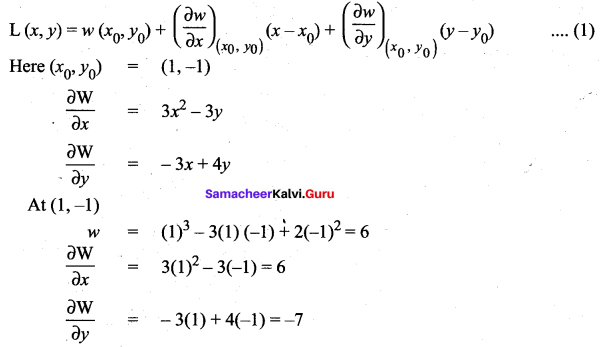(1) ⇒ Let(x, y) = 6 + 6(x – 1) – 7(y + 1)
= 6x – 6 – 7y – 7
= = 6x – 7y – 7

Question 2.
Let z(x, y) = x2y + 3xy4, x, y ∈ R, Find the linear approximation for z at (2, -1).
Solution: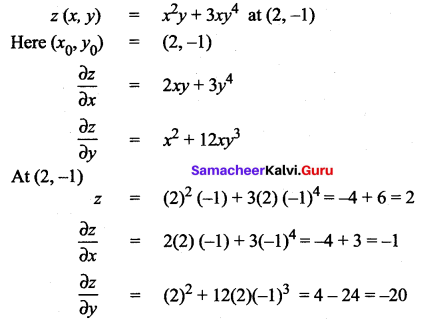Linear approximation is given by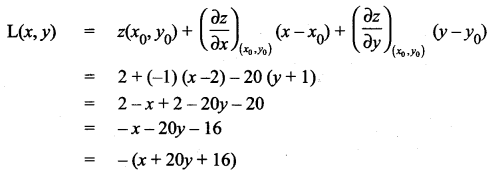Question 3.
If v(x, y) = x2 – xy + $$\frac{1}{4}$$y2 + 7, x, y ∈ R, find the differential dv.
Solution: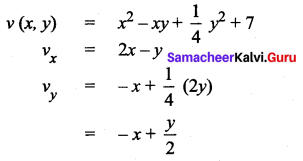The differential is dv = (2x -y) dx + (- x + $$\frac{y}{2}$$)dy

Question 4.
Let W(x, y, z) = x2 – xy + 3sinz,x,y, z ∈ R. Find the linear approximation at (2, -1, 0).
Solution:
w (x, y, z) = x2 – xy + 3 sin z
Here(x0, y0, y0) = (2, -1, 0)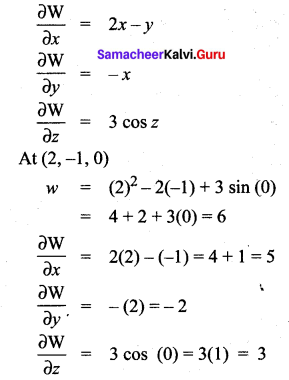Linear approximation is given by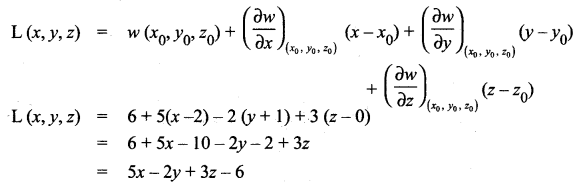Question 5.
Let V (x, y, z) = xy + yz + zx, x, y, z ∈ R. Find the differential dV.
Solution:
V(x, y, z) = xy + yz + zx
Vx = y + z
Vy = x + z
Vz = y + x
The differential is dV = (y + z) dx + (x + z) dy + (y + x) dz